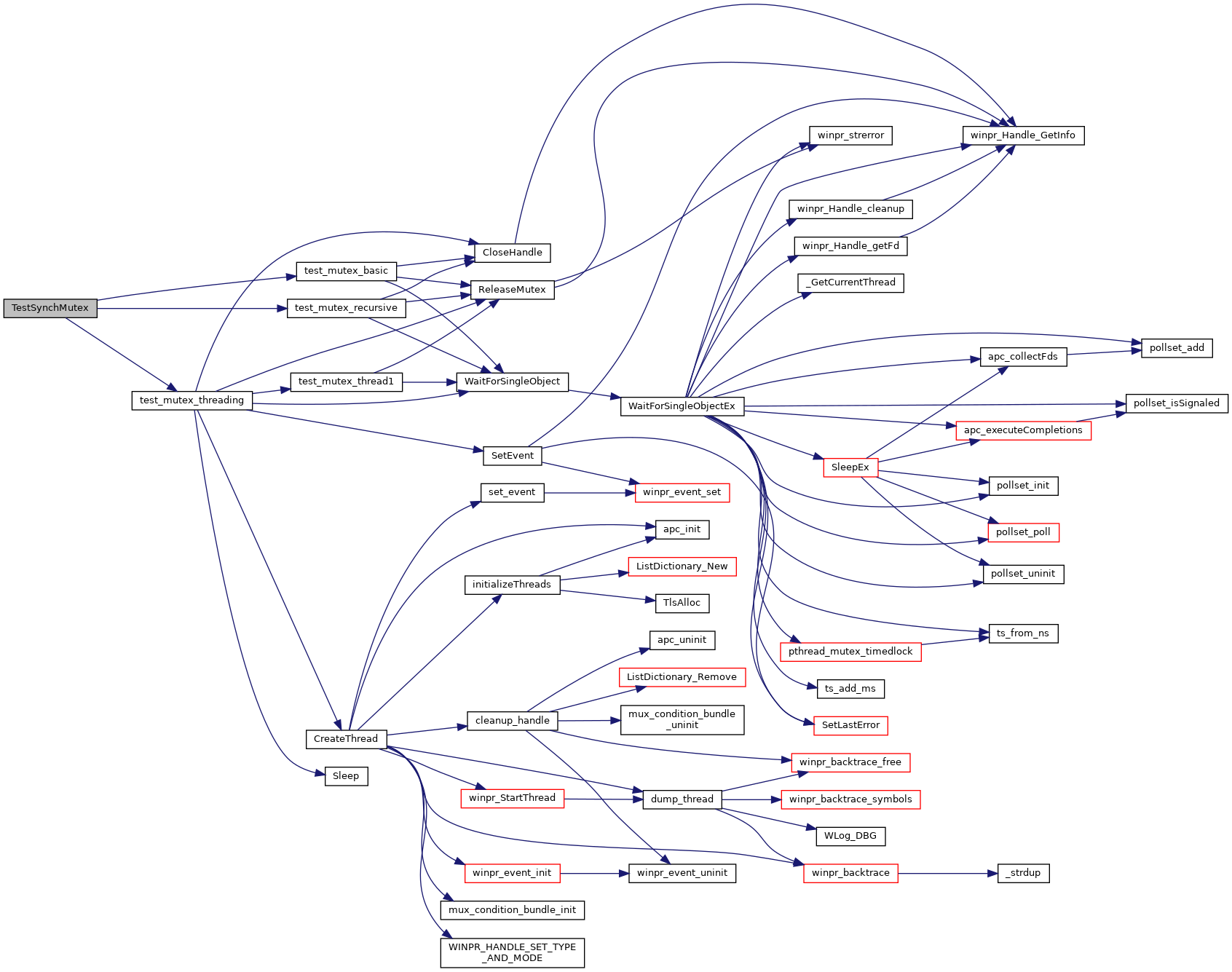FreeRDP
TestSynchMutex.c File Reference
`#include <winpr/crt.h>`
`#include <winpr/synch.h>`
`#include <winpr/thread.h>`

## Functions

static BOOL test_mutex_basic (void)

static BOOL test_mutex_recursive (void)

static DWORD WINAPI test_mutex_thread1 (LPVOID lpParam)

int TestSynchMutex (int argc, char *argv[])

## ◆ test_mutex_basic()

 static BOOL test_mutex_basic ( void )
static
Here is the call graph for this function: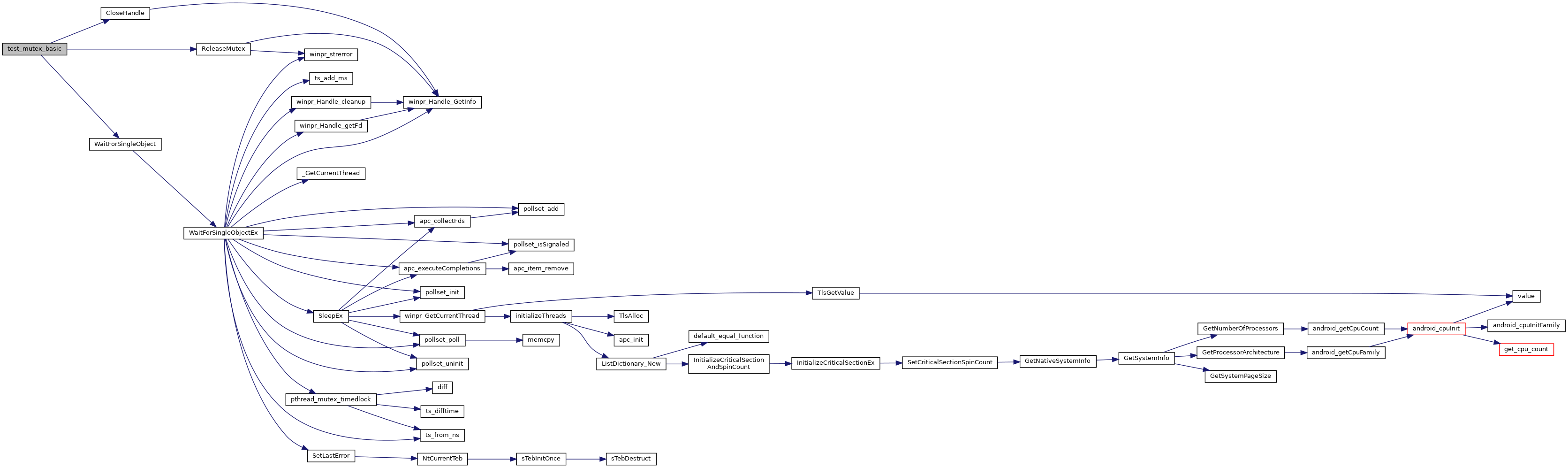Here is the caller graph for this function: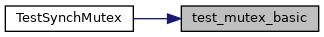## ◆ test_mutex_recursive()

 static BOOL test_mutex_recursive ( void )
static
Here is the call graph for this function: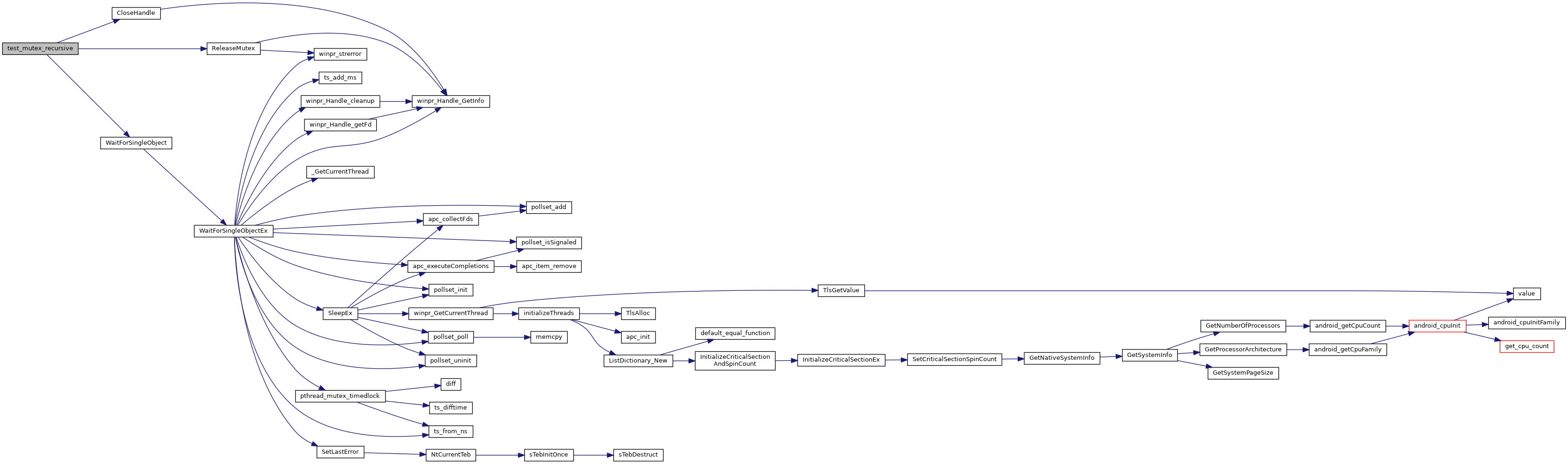Here is the caller graph for this function: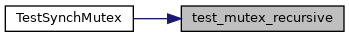static DWORD WINAPI test_mutex_thread1 ( LPVOID lpParam )
static

at this point: thread1_mutex1 is expected to be locked thread1_mutex2 is expected to be unlocked defined task: try to lock thread1_mutex1 (expected to fail) lock and unlock thread1_mutex2 (expected to work)

Here is the call graph for this function: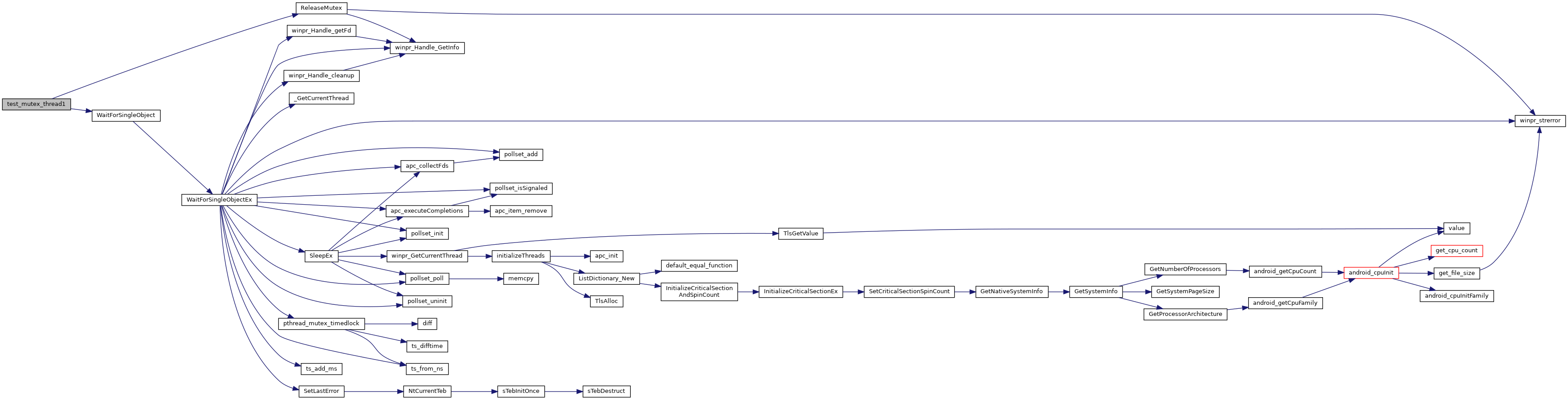Here is the caller graph for this function: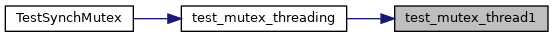static BOOL test_mutex_threading ( void )
static
Here is the call graph for this function: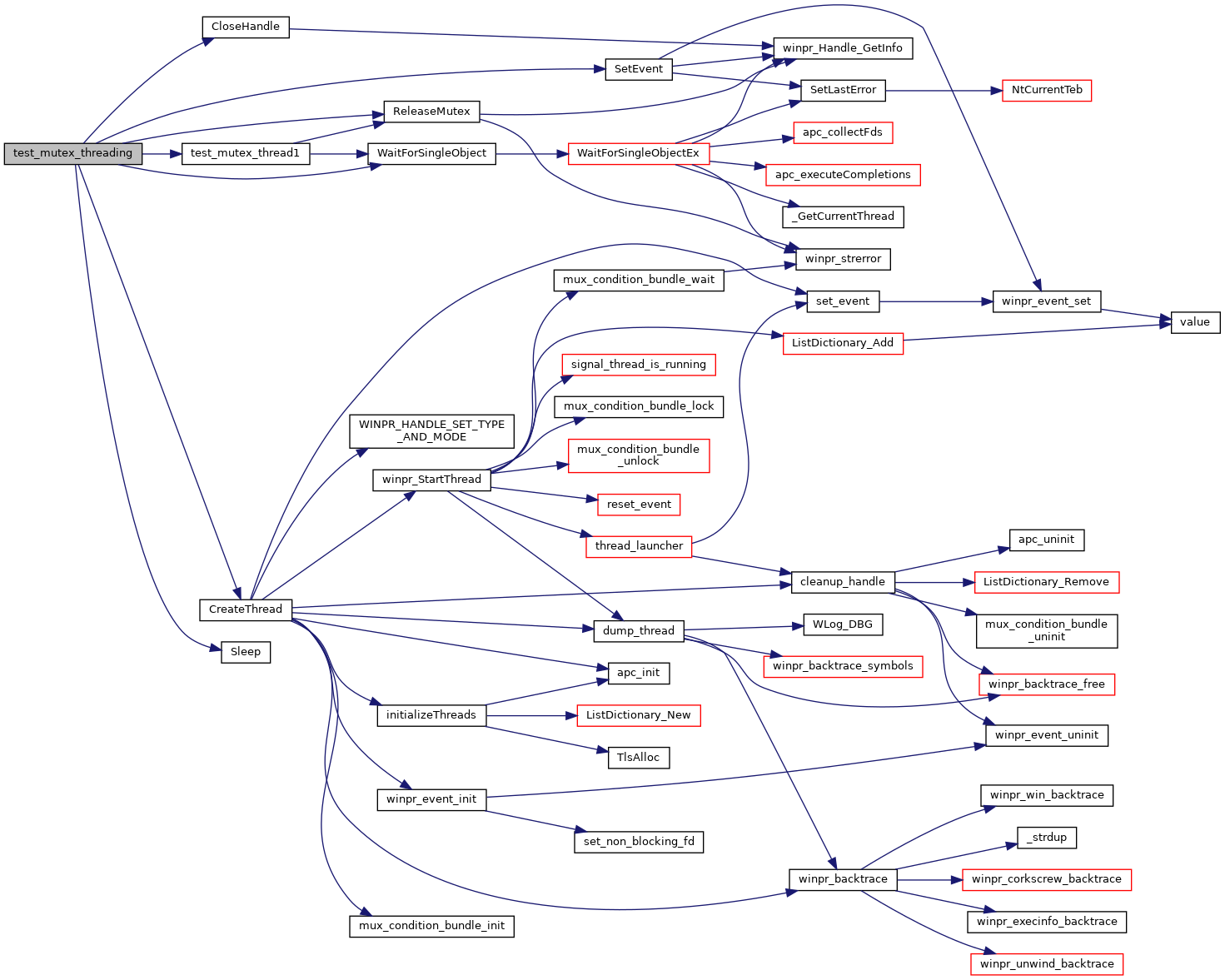Here is the caller graph for this function: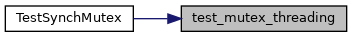## ◆ TestSynchMutex()

 int TestSynchMutex ( int argc, char * argv[] )
Here is the call graph for this function: